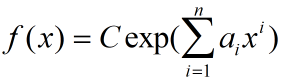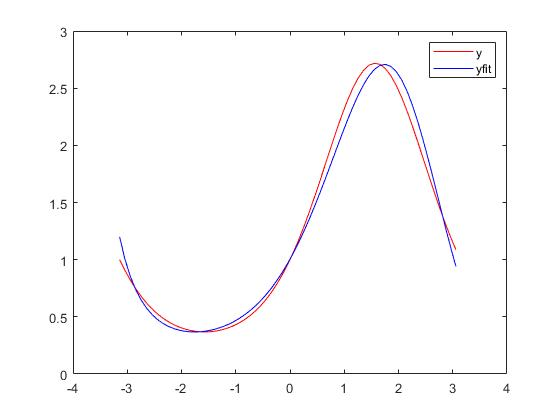2021-09-16 09:28

# 知道一组具体数值，如何求解对数据最优拟合的表达式• 好问题 提建议
• 收藏

#### 1条回答默认 最新

•joel_1993 2021-09-16 11:13
已采纳

你好，你这里还有指数啊，所以类似的，我也定义一个函数

``````x = -pi:0.1:pi;
y = exp(sin(x));
p = polyfit(x,log(y),3);%取n=3
yfit = exp(p(end))*exp(polyval([p(1:end-1),0],x));
plot(x,y,'r-', x,yfit,'b-')
legend('y','yfit')
``````已采纳该答案
评论
解决 无用
打赏 举报